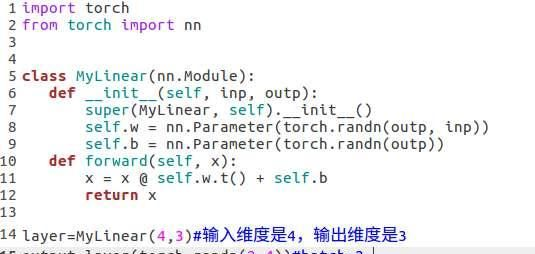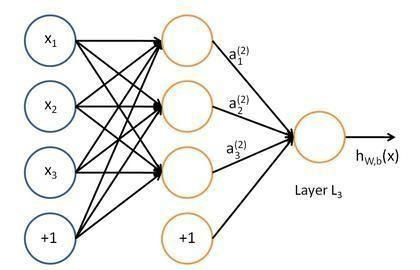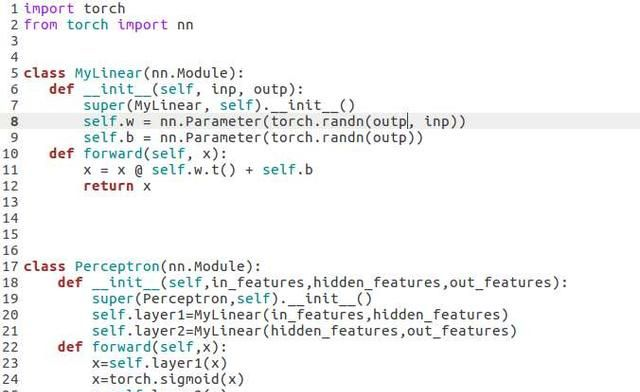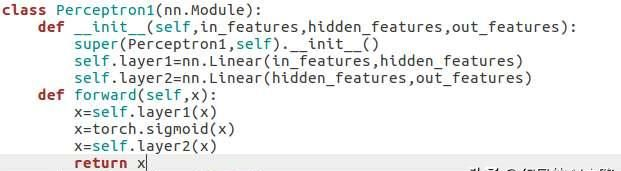# 每天五分钟深度学习框架pytorch：神经网络工具箱nn的介绍

2019年11月09日 15:39 吉运排球

torch.nn是专门为深度学习设计的工具箱，它的核心数据结构是Module类，它是一个抽象的概念，它既可以表示神经网络的一层，又可以表示一个包含很多层的神经网络。import torch from torch import nn class MyLinear(nn.Module): def __init__(self, inp, outp): super(MyLinear, self).__init__() self.w = nn.Parameter(torch.randn(outp, inp)) self.b = nn.Parameter(torch.randn(outp)) def forward(self, x): x = x @ self.w.t() + self.b return x layer=MyLinear(4,3)#输入维度是4，输出维度是3 output=layer(torch.randn(2,4))#batch=2class MyLinear(nn.Module): def __init__(self, inp, outp): super(MyLinear, self).__init__() self.w = nn.Parameter(torch.randn(outp, inp)) self.b = nn.Parameter(torch.randn(outp)) def forward(self, x): x = x @ self.w.t() + self.b return x class Perceptron(nn.Module): def __init__(self,in_features,hidden_features,out_features): super(Perceptron,self).__init__() self.layer1=MyLinear(in_features,hidden_features) self.layer2=MyLinear(hidden_features,out_features) def forward(self,x): x=self.layer1(x) x=torch.sigmoid(x) x=self.layer2(x) return x perceptron=Perceptron(3,4,1){!-- PGC_COLUMN --}

class Perceptron1(nn.Module): def __init__(self,in_features,hidden_features,out_features): super(Perceptron1,self).__init__() self.layer1=nn.Linear(in_features,hidden_features) self.layer2=nn.Linear(hidden_features,out_features) def forward(self,x): x=self.layer1(x) x=torch.sigmoid(x) x=self.layer2(x) return x perceptron=Perceptron1(3,4,1)